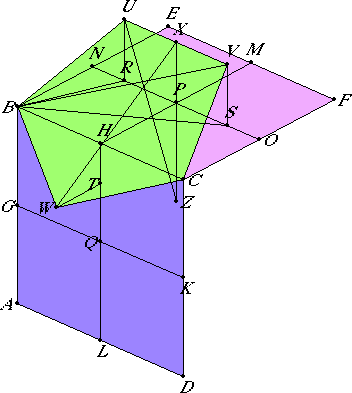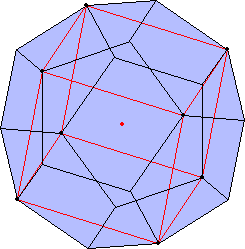# Proposition 17

To construct a dodecahedron and comprehend it in a sphere, like the aforesaid figures; and to prove that the square on the side of the dodecahedron is the irrational straight line called apotome.

Let ABCD and CBEF, two planes of the aforesaid cube at right angles to one another, be set out. Bisect the sides AB, BC, CD, DA, EF, EB, and FC at G, H, K, L, M, N, and O respectively, and join GK, HL, MH, and NO. Cut the straight lines NP, PO, and HQ in extreme and mean ratio at the points R, S, and T respectively, and let RP, PS, and TQ be their greater segments. Set up RU, SV, and TW from the points R, S, and T at right angles to the planes of the cube towards the outside of the cube, and make them equal to RP, PS, and TQ. Join UB, BW, WC, CV, and VU.

I say that the pentagon UBWCV is equilateral, in one plane, and equiangular.Join RB, SB, and VB.

XIII.4

Then, since the straight line NP is cut in extreme and mean ratio at R, and RP is the greater segment, therefore the sum of the squares on PN and NR is triple the square on RP.

But PN equals NB, and PR equals RU, therefore the sum of the squares on BN and NR is triple the square on RU.

I.47

But the square on BR equals the sum of the squares on BN and NR, therefore the square on BR is triple the square on RU. Hence the sum of the squares on BR and RU is quadruple the square on RU.

But the square on BU equals the sum of the squares on BR and RU, therefore the square on BU is quadruple the square on RU. Therefore BU is double RU.

But VU is also double UR, for SR is also double PR, that is, of RU, therefore BU equals UV.

Similarly it can be proved that each of the straight lines BW, WC, and CV also equals each of the straight lines BU and UV. Therefore the pentagon BUVCW is equilateral.

I say next that it is also in one plane.

I.31

Draw PX from P parallel to each of the straight lines RU and SV and toward the outside of the cube, and join XH and HW.

I say that XHW is a straight line.

Since HQ is cut in extreme and mean ratio at T, and QT is its greater segment, therefore HQ is to QT as QT is to TH. But HQ equals HP, and QT equals each of the straight lines TW and PX, therefore HP is to PX as WT is to TH.

XI.6

And HP is parallel to TW, for each of them is at right angles to the plane BD, and TH is parallel to PX, for each of them is at right angles to the plane BF.

VI.32

But if two triangles XPH and HTW, which have two sides proportional to two sides are placed together at one angle so that their corresponding sides are also parallel, then the remaining straight lines are in a straight line, therefore XH is in a straight line with HW.

XI.1

But every straight line is in one plane, therefore the pentagon UBWCV is in one plane.

I say next that it is also equiangular.

Since the straight line NP is cut in extreme and mean ratio at R, and PR is the greater segment, while PR equals PS, therefore NS is also cut in extreme and mean ratio at P, and NP is the greater segment. Therefore the sum of the squares on NS and SP is triple the square on NP.

But NP equals NB, and PS equals SV, therefore the squares on NS and SV is triple the square on NB. Hence the sum of the squares on VS, SN, and NB is quadruple the square on NB.

But the square on SB equals the sum of the squares on SN and NB, therefore the sum of the squares on BS and SV, that is, the square on BV, for the angle VSB is right, is quadruple the square on NB. Therefore VB is double BN.

But BC is also double BN, therefore BV equals BC.

I.8

And, since the two sides BU and UV equal the two sides BW and WC, and the base BV equals the base BC, therefore the angle BUV equals the angle BWC.

Similarly we can prove that the angle UVC also equals the angle BWC. Therefore the three angles BWC, BUV, and UVC equal one another.

XIII.7

But if in an equilateral pentagon three angles equal one another, then the pentagon is equiangular, therefore the pentagon BUVCW is equiangular.

And it was also proved equilateral, therefore the pentagon BUVCW is equilateral and equiangular, and it is on one side BC of the cube.

XI.Def.28

Therefore, if we make the same construction in the case of each of the twelve sides of the cube, a solid figure will be constructed which is contained by twelve equilateral and equiangular pentagons, and which is called a dodecahedron.

It is now required to comprehend it in the given sphere, and to prove that the side of the dodecahedron is the irrational straight line called apotome.

Produce XP, and let the produced straight line be XZ.

XI.38

Therefore PZ meets the diameter of the cube, and they bisect one another, for this has been proved in the next to the last theorem of the eleventh book.

Let them cut at Z. Therefore Z is the center of the sphere which comprehends the cube, and ZP is half of the side of the cube.

Join UZ.

XIII.4

Now, since the straight line NS is cut in extreme and mean ratio at P, and NP is its greater segment, therefore the sum of the squares on NS and SP is triple the square on NP.

But NS equals XZ, for NP also equals PZ, and XP equals PS.

But PS also equals XU, since it also equals RP. Therefore the sum of the squares on ZX and XU is triple the square on NP.

But the square on UZ equals the sum of the squares on ZX and XU, therefore the square on UZ is triple the square on NP.

XIII.15

But the square on the radius of the sphere which comprehends the cube is also triple the square on the half of the side of the cube, for it has previously been shown how to construct a cube and comprehend it in a sphere, and to prove that the square on the diameter of the sphere is triple the square on the side of the cube.

But, if the whole is so related to the whole as the half to the half also, and NP is half of the side of the cube, therefore UZ equals the radius of the sphere which comprehends the cube.

And Z is the center of the sphere which comprehends the cube, therefore the point U is on the surface of the sphere.

Similarly we can prove that each of the remaining angles of the dodecahedron is also on the surface of the sphere, therefore the dodecahedron has been comprehended in the given sphere.

I say next that the side of the dodecahedron is the irrational straight line called apotome.

Since, when NP is cut in extreme and mean ratio, RP is the greater segment, and, when PO is cut in extreme and mean ratio, PS is the greater segment, therefore, when the whole NO is cut in extreme and mean ratio, RS is the great er segment.

V.15

Thus, since NP is to PR as PR is to RN, the same is true of the doubles also, for parts have the same ratio as their equimultiples, therefore NO is to RS as RS is to the sum of NR and SO. But NO is greater than RS, therefore RS is also greater than the sum of NR and SO, therefore NO is cut in extreme and mean ratio, and RS is its greater segment.

But RS equals UV, therefore, when NO is cut in extreme and mean ratio, UV is the greater segment. And, since the diameter of the sphere is rational, and the square on it is triple the square on the side of the cube, therefore NO, being a side of the cube, is rational.

But if a rational line is cut in extreme and mean ratio, each of the segments is an irrational apotome.

XIII.6

Therefore UV, being a side of the dodecahedron, is an irrational apotome.

Q.E.F.

# Corollary

From this it is clear that when the side of the cube is cut in extreme and mean ratio, the greater segment is the side of the dodecahedron.

## Guide

#### Cubes and regular dodecahedra

Euclid’s construction of a dodecahedron is particularly easy because he circumscribed his dodecahedron about a cube. Just as a regular tetrahedron can be circumscribed by a cube, a cube can be circumscribed by a regular dodecahedron, indeed, two regular dodecahedra.

Also, each cube circumscribes two regular tetrahedra, and a regular dodecahedron circumscribes five cubes, and also ten tetrahedra.

#### Coordinates for the vertices of the dodecahedron

We can specify a coordinate system so that the center of the sphere is located at the origin and the eight vertices of the cube are located at

(1,1,1)   (1,1,–1)   (1,–1,1)   (1,–1,–1)   (–1,1,1)   (–1,1,–1)   (–1,–1,1)   (–1,–1,–1)The points A a through F in Euclid’s construction may be assigned six of these coordinates.

A = (–1,–1,–1), B = (–1,1,–1), C = (1,1,–1), D = (1,–1,–1), E = (–1,1,1), F = (1,1,1).

After bisecting the sides, the points G through Q receive the following coordinates.

G = (–1,0,–1), H = (0,1,–1), K = (1,0,–1), L = (0,–1,–1), M = (0,1,1),
N = (–1,1,0), O = (1,1,0), P = (0,1,0), Q = (0,0,–1).

The points R, S, and T cut the lines they’re on into extreme and mean ratios, so they have these coordinates:

R = (–x,1,0), S = (x,1,0), T = (0,x,–1),

where x equals (√5 – 1)/2. Finally, points U, V, and W are outside the original cube with the coordinates

U = (–x,1+x,0), V = (x,1+x,0), W = (0,x,–1–x),

#### Use of this construction

Constructing a dodecahedron is an end in itself. This construction and the corollary are also used in XIII.18 where the five regular polyhedra are compared.